## Slope calculator calculate slope [m], equation of straight line, distance between 2 points, midpoint and angle by input position of 2 points both on x-axis and y-axis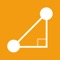# Slope Calculator Plus

by Nitrio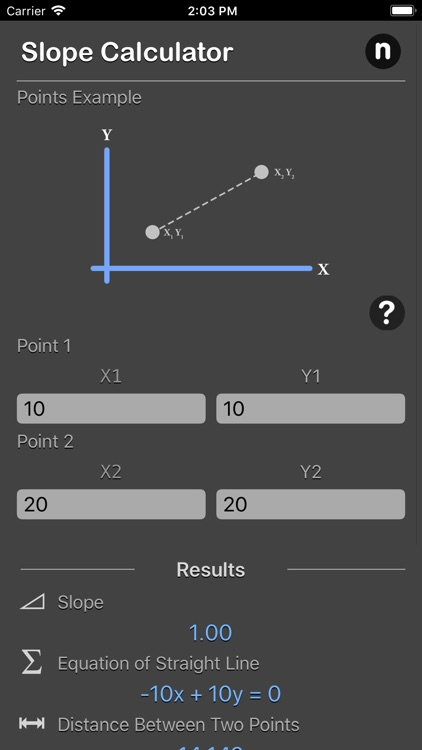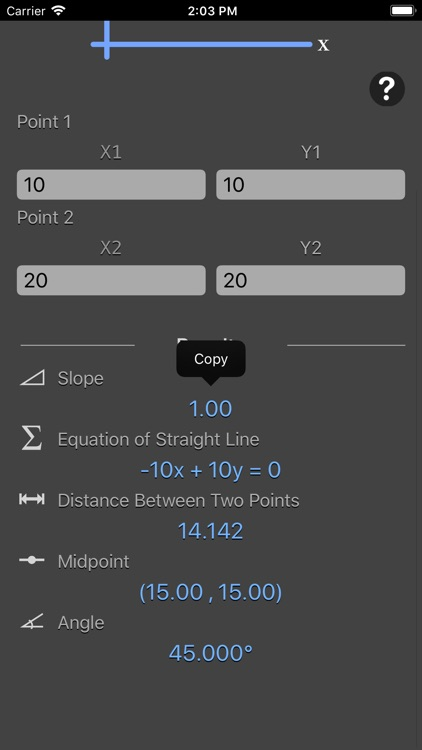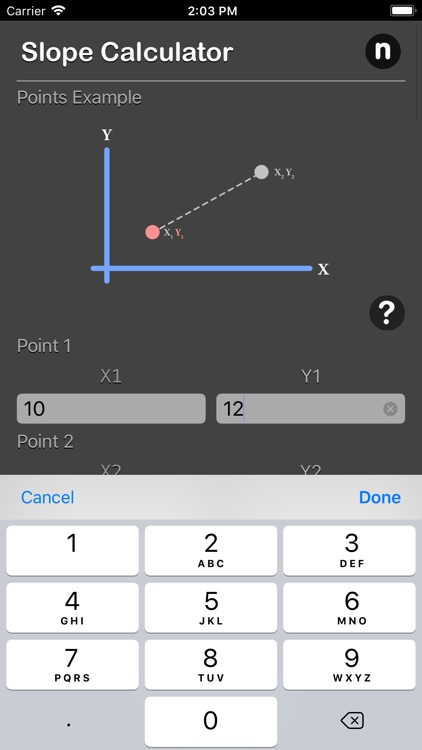Slope calculator calculate slope [m], equation of straight line, distance between 2 points, midpoint and angle by input position of 2 points both on x-axis and y-axis.### App Details

Version
1.0
Rating
(7)
Size
15Mb
Genre
Utilities Productivity
Last updated
April 30, 2018
Release date
April 30, 2018

### App Screenshots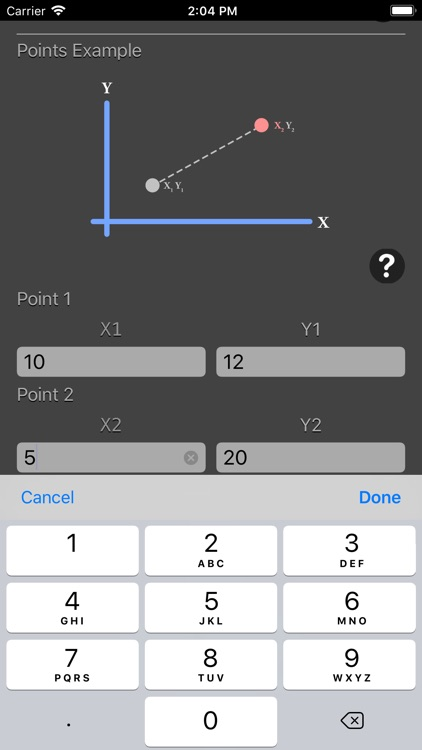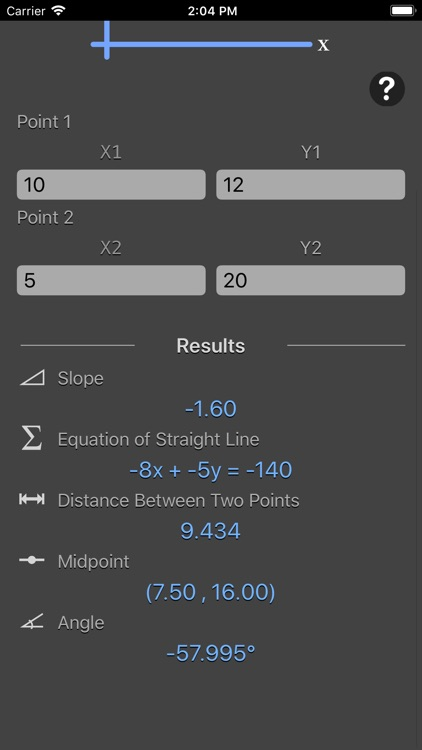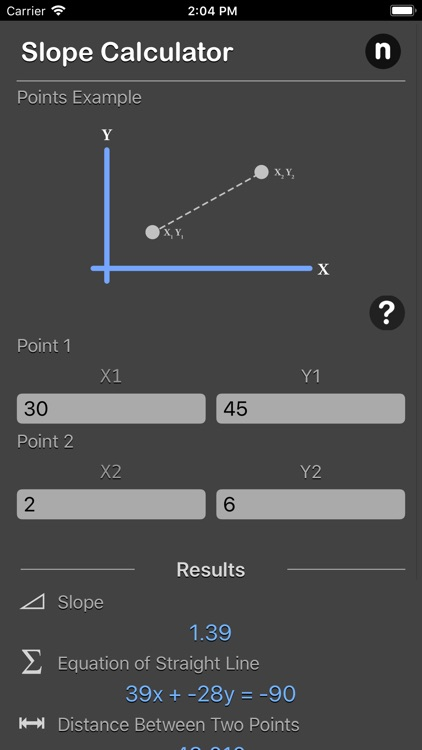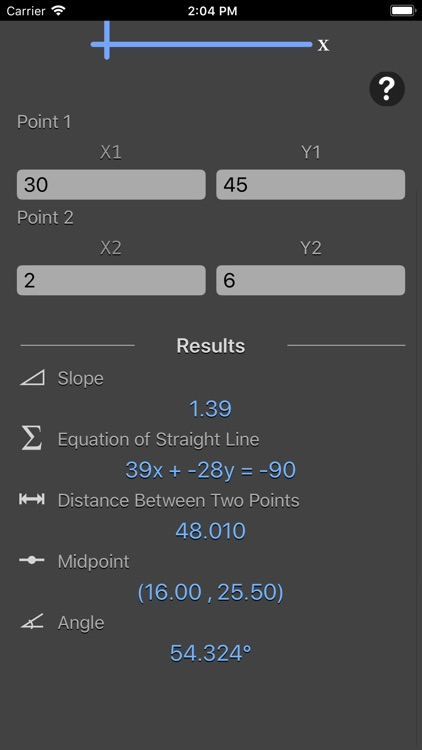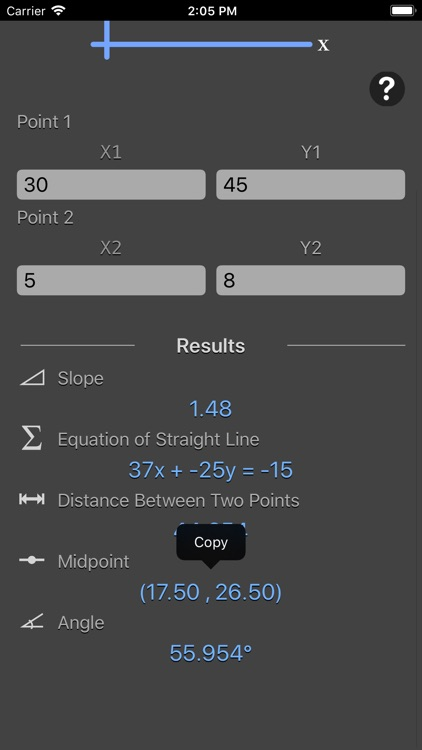### App Store Description

Slope calculator calculate slope [m], equation of straight line, distance between 2 points, midpoint and angle by input position of 2 points both on x-axis and y-axis.

Features:
- Calculate slope [m], equation of straight line, distance between 2 points, midpoint and angle by input position of 2 points both on x-axis and y-axis
- Instant result output
- Result are copy-able
- Reference image for better understanding on input point
- Formula are include for better understanding

Slope, sometimes referred to as gradient in mathematics, is a number that measures the steepness and direction of a line, or a section of a line connecting two points, and is usually denoted by m. Generally, a line's steepness is measured by the absolute value of its slope, m. The larger the value is, the steeper the line. Given m, it is possible to determine the direction of the line that m describes based on its sign and value:

- A line is increasing, and goes upwards from left to right when m > 0
- A line is decreasing, and goes downwards from left to right when m < 0
- A line has a constant slope, and is horizontal when m = 0
- A vertical line has an undefined slope, since it would result in a fraction with 0 as the denominator.

The slope is represented mathematically as:
m = (y2 - y1) / (x2 - x1)

Refer to the Pythagorean theorem we can calculate the distance provided in the calculator:
d = √(x2 - x1)2 + (y2 - y1)2

Given the slope (m), it is possible to find angle θ using the following equation:
θ = tan-1(m)

*This is a universal app that work for both iPhone and iPad.

Thanks for your support and do visit nitrio.com for more apps for your iOS devices.

Disclaimer:
AppAdvice does not own this application and only provides images and links contained in the iTunes Search API, to help our users find the best apps to download. If you are the developer of this app and would like your information removed, please send a request to [email protected] and your information will be removed.